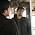Why Quicksort is better than Mergesort

This question was asked me in one of the interview and this is one of the unique questions among all the questions asked from me in all of my interviews. At that time, I couldn't answer this question.

After that, I searched on various technical forums to find out its answer. This question can also be asked like Both Quicksort and Mergesort have O(nlogn) time complexity, but most people use Quicksort instead of Mergesort. Firstly, lets find out:
• What is Quick Sort
• What is Merge Sort
Mostly all of the programmers know that quicksort is O(nlog n) on average and O(n2) in the worst case. and mergesort has O(nlogn).

What is Quick Sort

Quicksort is a divide and conquer algorithm. Firstly, it divides a large array into two smaller sub-array: the low elements and the high elements. and then recursively sort the sub-arrays. The steps involved in Quick sort are:
• Pick an element, called a pivot, from the array.
• Reorder the array so that all elements with values less than the pivot come before the pivot. While all elements with values greater than the pivot come after it. After this partitioning, the pivot is in its final position. This is called the partition operation.
• Recursively apply the above steps to the sub-array of elements with smaller values and separately to the sub-array of elements with greater values.

Example

public void quicksort(int arr[], int first, int last)
{
int i,j,pivot,temp;

if(first < last)
{
pivot = first;
i = first;
j = last;

while(i < j)
{
while(arr[i] <= arr[pivot] && i < last)
i++;
while(arr[j] > arr[pivot])
j--;
if(i < j)
{
temp = arr[i];
arr[i] = arr[j];
arr[j] = temp;
}
}

temp = arr[pivot];
arr[pivot] = arr[j];
arr[j] = temp;
quicksort(arr, first, j-1);
quicksort(arr, j+1, last);
}
}

What is Merge Sort

Merge sort is an O(n log n) comparison-based sorting algorithm. Most implementations produce a stable sort, meaning that the implementation preserves the input order of equal elements in the sorted output. It is a divide and conquer algorithm. A merge sort works as follows:
• Divide the unsorted list into n sublists, each containing 1 element.
• Repeatedly merge sublists to produce new sorted sublists until there is only 1 sublist remaining. This will be the sorted list.

Example

public void merge(int a[], int low, int mid, int high)
{
int b[] = new int[a.length];
int i = low, j = mid + 1, k = 0;

while (i <= mid && j <= high) {
if (a[i] <= a[j])
b[k++] = a[i++];
else
b[k++] = a[j++];
}
while (i <= mid)
b[k++] = a[i++];

while (j <= high)
b[k++] = a[j++];

k--;
while (k >= 0) {
a[low + k] = b[k];
k--;
}
}

void mergesort(int a[], int low, int high)
{
if (low < high) {
int m = (high + low)/2;
mergesort(a, low, m);
mergesort(a, m + 1, high);
merge(a, low, m, high);
}
}

Why Quick sort is better than Merge sort

Quicksort has O(n2) worst-case runtime and O(nlogn) average case runtime while mergesort has O(nlogn) time complexity. But, the comparison is completely about constant factors. In particular, the choice is to select an optimal pivot for Quicksort versus the copy of the entire input for Mergesort. It turns out that the former is more efficient but there is no theory behind this.

Note that Quicksort will make more recursive calls, but allocating stack space is cheap (almost free in fact, as long as you don't blow the stack) and you re-use it. Allocating a giant block on the heap is quite a bit more expensive, but both are O(logn) overheads.

Lastly, Quicksort is slightly sensitive to input that happens to be in the right order, in which case it can skip some swaps. Mergesort doesn't have any such optimizations, which also makes Quicksort a bit faster compared to Mergesort. Below is the comparison graph of Quick sort, heap sort and merge sort.
Above figure shows the timing results for all three sorting programs in terms of the execution time per key, where a key is an element in the sorting array.

Conclusion

But, there is no sorting algorithm which is always optimal. You should choose whichever one suits your needs. If you need an algorithm that is the quickest for most cases, and might be slow in some other cases, and you don't need a stable sort.

1.2.